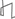Technical Reports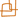Work Products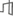Research Abstracts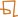Historical Collections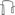Research Abstracts - 2006IntroductionLanguage, Learning, Vision & GraphicsPhysical, Biological & Social SystemsSystemsTheory### Trace Norm Clustering

#### Jason D. M. Rennie, John D. Barnett & Tommi S. Jaakkola

##### Introduction

We are developing clustering algorithms that favor clusters of low dimension rather than small radius. Each cluster, a subset of rows of the data matrix, is modeled with a trace norm distribution over matrices. The trace norm distribution provides a smooth penalty on the effective dimensionality of the clusters. The use of the trace norm distribution requires us to evaluate a normalization constant that involves an integral over penalized singular values, modulated by the volume of a scaled Stiefel manifold. The calculation can be done in closed form for small matrices; for larger matrices we provide an efficient variational approximation. We illustrate the exact clustering algorithm in simple cases and provide an efficient search algorithm for partitioning large data matrices. Our algorithm has the potential for wide application, including areas as diverse as textual topic segmentation and biological inference.

##### Main Idea

Our work is an extension of Maximum Margin Matrix Factorization (MMMF) . MMMF is a general framework for learning a reduced dimensionality representation of a data set for a particular supervised task (such as classification or regression). It has been successfully applied to collaborative prediction , significantly out-performing all nine algorithms against which it was compared. MMMF minimizes a weighted sum of data loss plus a regularlization term. An advantage of MMMF compared to other methods is that it uses the trace norm of the parameter matrix for regularization. The trace norm is the sum of (absolute values of) singular values of a matrix. Similar to the way the L1-norm encourages feature selection for classification, the trace norm encourages a low-rank solution. Furthermore, as long as the data loss is a convex function of the parameters, minimization of the MMMF objective is a well-posed problem---optimization will find the unique, global minimum.

We introduce a new distribution based on the trace norm, what we call the ``trace norm distribution.'' This is a distribution over matrices, and hence can be used to model an entire data set (one datum per row) or a parameter matrix that is used to model the data set. Normalization of this distribution is not trivial. We must do a change of variables to the the Singular Value Decomposition. The form of the SVD Jacobian makes evaluation difficult. We can evaluate the integral exactly for small matrices. For larger matrices, exact computation is impossible. One aspect of our work is the development of approximation algorithms for the normalization constant; we have developed two techniques so far---one variational approximation and one based on Monte Carlo.

We treat the clustering problem as inference where we assume that each datum is generated from a trace norm distribution. All data from a single cluster is generated jointly from a trace norm distribution; data for each cluster is generated independently. The normalization constant provides the necessary trade-off factor so that the best solution is one where data (or corresponding parameters) between clusters is largely orthogonal and rank within each cluster is low.

##### Related Work

At its core, clustering requires a notion of similarity between points. In many algorithms, including spectral clustering [3,4] and k-means, Euclidean distance provides the basis for such a measure. In contrast to these approaches, trace norm clustering implicitly uses a combination of cluster volume and dimension as a similarity measure, based on the trace norm distribution. Dimensionality reduction techniques seek to find a low rank projection of the data; PCA, in particular, has been used for clustering in the gene-shaving algorithm , a top-down technique that splits clusters based on the correlations between each point and the principal components. Our technique is similar in its use of singular values, but essentially groups points that can have multiple interacting factors. In this sense, it is similar to sparse PCA , where an L1 penalty is applied to the loadings to yield a sparse matrix, with each point having only a subset of factors with nonzero weights. Using the trace norm, however, aims to have sparse singular values rather than sparse coefficients, thus keeping the dimensionality of clusters low.

##### Clustering Intuition

Clustering aims to partition the data in such a way that points within a cluster are similar, but the clusters themselves are dissimilar. Often, Euclidean distance is used as a dissimilarity measure; here, instead of looking for clusters with low distance between the points they include, we aim to find clusters of low dimension. This may be appropriate for data where each cluster arises through the interaction of a few linear processes. Rather than using an explicit rank constraint, we can use the trace norm as a measure of cluster tightness. The fact that trace norm regularization prefers sparse singular values, means that we should find subspaces that are flat (low in dimension) rather than small in radius.

If we simply find the partitioning to minimize the trace norm, we would always place all of the points into a single cluster, as the combined trace norms of any split is at least the trace norm of the original matrix. However, if we view clustering in a probabilistic setting, then the normalizing constant of the distribution balances the trace norm, and no size clustering is preferred a priori. In fact, for data that is cleanly arranged into orthogonal sub-spaces, our algorithm will always find the correct partitioning. For real-world, noisy data, we use a single paramter to control between the desire for a coarse or fine clustering.

##### Probabilistic Framework

We assume that the data (or parameters of the data) is in the form of a real matrix, one datum per row. Furthermore, we assume that each datum was generated via one of many independent, low-rank processes. We would like to recover this structure by finding a clustering of the data that respects this structure as closely as possible. We do this by assuming that the data is partitioned and that the data from each partition is generated from a trace norm distribution (below). We recover the clusters by finding the partitioning of data points that best fits our trace norm model.

We define the trace norm distribution of a fixed-size matrix, X ∈ ℜm × n as proportional to the exponentiated negative trace norm, with a single parameter, λ, dictating the ``width'' of the distribution,

Pm × n(X|λ) ∝ exp(-λ ||X||Σ).

For clustering, we assume that clusters are independent of one another in how they generate data. And, the data from a single cluster is generated according to a trace norm distribution. Points from a single cluster will tend to be somewhat ``flat,'' with just a few large singular values.

We perform inference using the partition model by determining the partitioning for a set of unlabeled data that yields the largest likelihood. In principle, this is simple: iterate over all possible partitionings, evaluating the data likelihood for each. However, there are (at least) exponentially many partitionings as a function of the number of data points; we are exploring efficient approximation methods for finding of the optimal partitioning.

##### Discussion

We have introduced a new framework for clustering based on associating points which lie together in a low-dimensional space. We use the trace norm as our basis, which provides a smooth penalty on the dimensionality of data. Incorporation of the trace norm into a distribution allowed us to compare partitionings of various sizes. Normalization of this distrubtion is computationally difficult, so we have developed approximation methods that would be useful for clustering of large data sets. We are experimenting with incremental and bottom-up clustering algorithms. We are in the process of applying this to text and biological data.

##### Acknowledgments

This project is supported in part by DARPA CALO project and in part by NIH grant GM68762.

##### References:

 Nathan Srebro, Jason D. M. Rennie and Tommi S. Jaakkola. Maximum-Margin Matrix Factorization. Advances in Neural Information Processing Systems 17. 2005.

 Jason D. M. Rennie and Nathan Srebro. Fast Maximum Margin Matrix Factorization for Collaborative Prediction. Proceedings of the 22nd International Conference on Machine Learning. 2005.

 Yair Weiss. Segmentation using eigenvectors: A unifying view. International Conference on Computer Vision. 1999.

 Andrew Y. Ng and Michael I. Jordan and Yair Weiss. On Spectral Clustering: Analysis and an algorithm. Advances in Neural Information Processing Systems 14. 2002.

 Trevor Hastie, Robert Tibshirani, Michael B Eisen, Ash Alizadeh, Ronald Levy, Louis Staudt, Wing C Chan, David Botstein and Patrick Brown. Gene shaving as a method for identifying distinct sets of genes with similar expression patterns. Genome Biology, 1, 1-21. 2000.

 Hui Zou, Trevor Hastie and Rob Tibshirani. Sparse Principal Component Analysis. Technical Report, Statistics Department, Stanford University. 2004.Computer Science and Artificial Intelligence Laboratory (CSAIL) The Stata Center, Building 32 - 32 Vassar Street - Cambridge, MA 02139 - USA tel:+1-617-253-0073 - publications@csail.mit.edu